# GSEB Solutions Class 8 Maths Chapter 8 Comparing Quantities Ex 8.3

Gujarat Board GSEB Textbook Solutions Class 8 Maths Chapter 8 Comparing Quantities Ex 8.3 Textbook Questions and Answers.

## Gujarat Board Textbook Solutions Class 8 Maths Chapter 8 Comparing Quantities Ex 8.3

Question 1.
Calculate the amount and compound interest on
(a) ₹ 10,800 for 3 years at 12 $$\frac{1}{2}$$ % per annum compounded annually.
(b) ₹ 18,000 for 2 $$\frac{1}{2}$$ years at 10% per annum compounded annually.
(c) ₹ 62,500 for 1 $$\frac{1}{2}$$ years at 8% per annum compounded half yearly.
(d) ₹ 8,000 for 1 year at 9% per annum compounded half yearly.
(You could use the year by year calculation using SI formula to verify.)
(e) ₹ 10,000 for 1 year at 8% per annum compounded half yearly.
Solution:
(a) Here, P = ₹ 10800, T = 3 years,
R = 12 $$\frac{1}{2}$$% p.a.
We have: A = P[1 + $$\frac{R}{100}$$]n
= $$₹ 10800\left[1+\frac{12 \frac{1}{2}}{100}\right]^{3}$$
[∵ Interest compound annually, ∴n = 3]
= ₹ 10800 $$\frac{225}{100}$$3
= ₹ 10800 × $$\frac{225}{100}$$ × $$\frac{225}{100}$$ × $$\frac{225}{100}$$
= ₹ $$\frac{675×9×9×9}{4×8}$$ = ₹ $$\frac{492075}{32}$$
∴ Amount = ₹ 15377.34
Now, compound interest
= ₹ 15377.34 – ₹ 10800 = ₹ 4577.34

(b) Here, P = ₹ 18000, T = 2$$\frac{1}{2}$$years,
R = 10% p.a.
∵ Interest is compounded annually,
n = 2 + $$\frac{1}{2}$$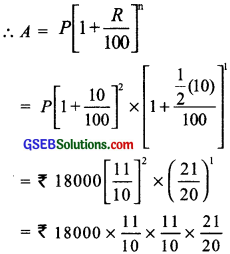= ₹ 9 × 11 × 11 × 21 = ₹ 22869
∴ Amount = ₹ 22869
CI = ₹ 22869 – ₹ 18000 = ₹ 4869

(c) Here P = ₹ 62500, T = 1$$\frac{1}{2}$$ r = 8% p.a.
Compounded half yearly,
R = 8% p.a. = 4% per half year
T = 1$$\frac{1}{2}$$year → n = 3 half years.
∴ Amount = P(1 + $$\frac{R}{100}$$)n
= ₹ 62500 (1 + $$\frac{4}{100}$$)3 = ₹ 62500 ($$\frac{26}{25}$$)3
= ₹ 62500 × $$\frac{26}{25}$$ × $$\frac{26}{25}$$ × $$\frac{26}{25}$$
= ₹ 4 × 26 × 26 26 = ₹ 70304
Amount = ₹ 70304
CI = ₹ 70304 – ₹ 62500 = ₹ 7804

(d) Here, P = ₹ 8000, T = 1 year, R = 9% p.a.
Interest is compounded half yearly,
∴ T = 1 year = 2 half years
R = 9% p.a. = $$\frac{9}{2}$$% half yearly
∴ Amount = P(1 + $$\frac{R}{100}$$)n
= ₹ 8000 × (1 + $$\frac{9}{200}$$)2
= ₹ 8000 × $$\frac{209}{200}$$ × $$\frac{209}{200}$$ = ₹ $$\frac{2 × 209 × 209}{10}$$
= ₹ $$\frac{87362}{10}$$ = ₹ 8736.20
CI = ₹ 8736.20 – ₹ 8000 = ₹ 736.20

(e) Here, P = ₹ 10000, T = 1 year
R = 8% p.a. compunded half yearly.
∴ R = 8% p.a. = 4% per half yearly
T = 1 year → n = 2 × 1 = 2
Now, amount = P(1 + $$\frac{R}{100}$$)n
= ₹ 10,000 (1 + $$\frac{4}{100}$$)2 = ₹ 10,000 ($$\frac{26}{25}$$)2
= ₹ 10,000 × $$\frac{26}{25}$$ × $$\frac{26}{25}$$
CI = ₹ 10816 – ₹ 10000 = ₹ 816.Question 2.
Kamala borrowed ₹ 26,400 from a Bank to buy a scooter at a rate of 15% p.a. compounded yearly What amount will she pay at the end of 2 years and 4 months to clear the loan?
(Hint: Find A for 2 years with interest is compounded yearly and then find SI on the 2nd year for $$\frac{4}{12}$$ years.)
Solution:
Note: Here, we shall calculate the amount for 2 years using the CI formula. Then this amount will become the principal for next 4 months, i.e., $$\frac{4}{12}$$ years.
Here, P = ₹ 26400, T = 2 years, R = 15% p.a
A = P[1 + $$\frac{R}{100}$$]n = ₹ 26400[1 + $$\frac{15}{100}$$]2
= ₹ 26400 × $$\frac{23}{20}$$2 = ₹ 26400 × $$\frac{23}{20}$$ × $$\frac{23}{20}$$
= ₹ 66 × 23 × 23 = ₹ 34914
Again, P = 34914. T = 4 months = $$\frac{4}{12}$$ years,
R = 15% p.a.
∴ Using, SI = $$\frac{P×R×T}{100}$$, we have
SI = ₹ $$\frac{5819×3}{10}$$ = ₹ $$\frac{17457}{10}$$ = ₹ 1745.70
Amount = SI + P
= ₹ (1745.70 + 34914) = ₹ 36659.70
Thus, the required amount to be paid to the bank after 2 years 4 month = ₹ 36659.70

Question 3.
Fabina borrows 12,500 at 12% per annum for 3 years al simple interest and Radha borrows the same amount for the same time period at 10% per annum, compounded annually. Who pays more interest and by how much?
Solution:
For Fabina
P = ₹ 12500, T = 3 years, R = 12% p.a.
∴ SI = $$\frac{P×R×T}{100}$$ = ₹ $$\frac{12500×3×12}{100}$$
= ₹ 125 × 3 × 12 = ₹ 4500
For Radha P = ₹ 12500, T = 3 years
R = 10% p.a. (Compounded annually)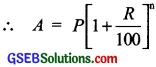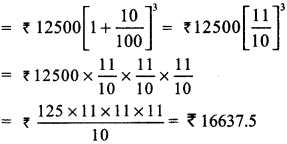∴ CI = ₹ 16637.5 – ₹ 12500 = ₹ 4137.50
Difference = ₹ 4500 – ₹ 4137.50 = ₹ 362.50
Thus, Fabina pays ₹ 362. 50 more.Question 4.
I borrowed ₹ 12,000 from Jamshed at 6% per annum simple interest for 2 years. Had I borrowed this sum at 6% per annum compound interest, what extra amount would I have to pay?
Solution:
For SI
Principal = ₹ 12000; Time = 2 years
Rate = 6% p.a.
SI = $$\frac{P×R×T}{100}$$ = ₹ $$\frac{12000×2×6}{100}$$
= ₹ (120 × 2 × 6) = ₹ 1440
For CI
Principal = ₹ 12000; Time = 2 years
Rate = 6% p.a.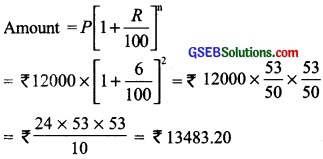∴ CI = ₹ 13483. 20 – ₹ 12000 = ₹ 1483.20
Thus, excess amount = ₹ 1483.20 – ₹ 1440 = ₹ 43.20

Question 5.
Vasudevan invested ₹ 60,000 at an interest rate of 12% per annum compounded half yearly. What amount would he get
(i) after 6 months?
(ii) after 1 year?
Solution:
(i) Amount after 6 months
P = ₹ 60000, T = $$\frac{1}{2}$$ year, n = 1
[∵ Interest is compounded half yearly]
R = 12% p.a. = 6% per half year
∴ Amount = P[1 + $$\frac{R}{100}$$]n
= ₹ 6000 [1 + $$\frac{6}{100}$$]1 = ₹ 60000 × $$\frac{53}{50}$$
= ₹ 1200 × 53 = ₹ 63600

(ii) Amount after 1 year
P = ₹ 60000
T = 1 year; n = 2
R = 12% p.a. = 6% per half year
∴ Amount = P[1 + $$\frac{R}{100}$$]n
= ₹ 60000 × [1 + $$\frac{6}{100}$$]2
= ₹ 60000 × $$\frac{53}{50}$$ × $$\frac{53}{50}$$ = ₹ 24 × 53 × 53
= ₹ 67416
Thus, amount after 6 months = ₹ 63600 and amount after 1 year = ₹ 67416Question 6.
Arif took a loan of ₹ 80,000 from a bank. If the rate of interest is 10% per annum, find the difference in amounts he would be paying after 1$$\frac{1}{2}$$ years if the interest is
(i) Compounded annually.
(ii) Compounded half yearly.
Solution:
(i) Compounded annually
P = 80000 R = 10% p.a.
T = $$\frac{1}{2}$$ years ⇒ n = 1 + $$\frac{1}{2}$$ T = 1$$\frac{1}{2}$$ years
Amount for 1st year.
A = P[1 + $$\frac{R}{100}$$]n = ₹ 80000 × [1 + $$\frac{10}{100}$$]1
= ₹ 80000 × $$\frac{10}{100}$$ × $$\frac{1}{2}$$ = ₹ 88000
SI on ₹ 88000 for next $$\frac{1}{2}$$ year
= ₹ 88000 × $$\frac{10}{100}$$ × $$\frac{1}{2}$$ = ₹ 440 × 10 = ₹ 4400
∴ Amount = ₹ 88000 + ₹ 44000 = ₹ 92400

(ii) Compounded half yearly
P = ₹ 80000 P = ₹ 80000
R = 10% p.a.
R = 10% p.a. = 5% per half year
T = 1$$\frac{1}{2}$$ years ⇒ n = 1 + $$\frac{1}{2}$$ T = 1$$\frac{1}{2}$$ years
⇒ n = 3
Amount for 1st year.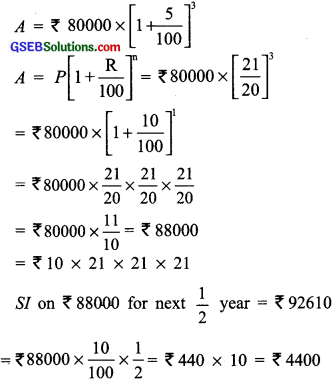∴ Amount = ₹ 88000 + ₹ 44000 = ₹ 92400
Thus, the difference between the two amounts = ₹ 92610 – ₹ 92400 = ₹ 210

Question 7.
Maria invested ₹ 8,000 in a business. She would be paid interest at 5% per annum compounded annually. Find:
(i) The amount credited against her name at the end of the second year.
(ii) The interest for the 3rd year.
Solution:
Principal = ₹ 8000
Rate = 5% p.a. compounded annually
(i) Time = 2 years ⇒ n = 2
∴ Amount = P[1 + $$\frac{R}{100}$$]n
= ₹ 8000[1 + $$\frac{5}{100}$$]2 = ₹ 8000 × $$\left[\frac{21}{20}\right]^{2}$$
= ₹ 8000 × $$\frac{21}{20}$$ × $$\frac{21}{20}$$ = ₹ (20 × 21 × 21)
= ₹ 8820
∴ Amount credited against her name at the end of the two years = ₹ 8820

(ii) Time = 3 years ⇒ n = 3
∴ Amount = ₹ 8000[1 + $$\frac{5}{100}$$]3
= ₹ 8000 $$\left[\frac{21}{20}\right]^{3}$$ = ₹ 8000 × $$\frac{21}{20}$$ × $$\frac{21}{20}$$ × $$\frac{21}{20}$$
= ₹ (21 × 21 × 21) = ₹ 9261
∵ Interest paid during 3rd year
= [Amount at the end of 3rd year] – [Amount at the end of 2nd year]
= ₹ 9261 – ₹ 8820 = ₹ 441Question 8.
Find the amount and the compound interest on ₹ 10,000 for 1$$\frac{1}{2}$$ years at 10% per annum, compounded half yearly. Would this interest be more than the interest he would get if it was compounded annually?
Solution:
Principal = ₹ 10,000 Time = 1$$\frac{1}{2}$$%
Rate = 10% p.a.

Case I:
Interest is compounded half yearly
We have r = 10 p.a. = 5% per half yearly
T = 1$$\frac{1}{2}$$ years ⇒ n = 3
∴ A = P[1 + $$\frac{R}{100}$$]n = ₹ 10000[1 + $$\frac{5}{100}$$]3
= ₹ $$\frac{5×21×21×21}{4}$$ = ₹ $$\frac{46305}{4}$$ = ₹ 11576.25
∴ Amount = ₹ 11576.25
Now CI = Amount – Principal
= ₹ 11576.25 – ₹ 10,000 = ₹ 1576.25

Case II:
Interest is compounded annually
We have R = 10% p.a. T = 1$$\frac{1}{2}$$year
Amount for 1 year = P[1 + $$\frac{R}{100}$$]n
= ₹ 10000 × [1 + $$\frac{10}{100}$$]1 = ₹ 10000 × $$\frac{11}{10}$$
= ₹ 11000
∴ Interest for 1st year = ₹ 11000 – ₹ 10,000
= ₹ 1000
∴ Interest for next $$\frac{1}{2}$$year on ₹ 11000
= $$\frac{P×R×T}{100}$$ = ₹ 11000 × $$\frac{10}{100}$$ × $$\frac{1}{2}$$ = ₹ 550
∴ Total interest = ₹ 1000 + ₹ 550 = ₹ 1550
Since ₹ 1576.25 > ₹ 1550
∴ Interest would be more in case if it is compounded half yearly.Question 9.
Find the amount which Ram will get on ₹ 4096, if he gave it for 18 months at 12$$\frac{1}{2}$$% per annum, interest being compounded half yearly?
Solution:
We have P = ₹ 4096 T = 18 months
R = 12$$\frac{1}{2}$$% p.a.
∵ Interest is compounded half yearly.
T = 18 months ⇒ n = $$\frac{18}{6}$$ = 3 six months
R = 12$$\frac{1}{2}$$% p.a. ⇒ R = (12$$\frac{1}{2}$$ ÷ 2)% per half year.
= ($$\frac{25}{2}$$ × $$\frac{1}{2}$$)% = $$\frac{25}{4}$$% half yearly
Now, A = P[1 + $$\frac{R}{100}$$]n = ₹ 4096 × [1 + $$\frac{25}{100}$$]3
= ₹ 4096 × $$\frac{17}{16}$$3 = ₹ 4096 × $$\frac{17}{16}$$ × $$\frac{17}{16}$$ × $$\frac{17}{16}$$
= ₹ (17 × 17 × 17) = ₹ 4913
Thus, the required amount = ₹ 4913

Question 10.
The population of a place increased to 54,000 in 2003 at a rate of 5% per annum?
(i) Find the population in 2001.
(ii) What would be its population is 2005?
Solution:
Population in 2003 is P = 54000
(i) Let the population in 2001 (i.e., 2 years ago) be P
Since rate of increment in population = 5% p.a.
∴ Present population = P(1 + $$\frac{5}{100}$$]2
or 54000 = P($$\frac{21}{20}$$]2 or 5400 = P($$\frac{441}{400}$$)
or P = $$\frac{54000 × 400}{441}$$ = 48979.59
= 48980 (approx)
Thus, the population in 2001 was about 48980

(ii) Initial population (in 2003). i.e., P = 54000
Rate of increment in population = 5% pa.
Time = 2 years ⇒ n = 2
∴ A = P[1 + $$\frac{R}{100}$$]2 = 54000 × $$\frac{21}{20}$$ × $$\frac{21}{20}$$
= 135 × 21 × 21 = 59535
Thus, the population in 2005 = 59535.Question 11.
In a laboratory. the count of bacteria in a certain experiment was increasing at the rate of 2.5% per hour. Find the bacteria at the end of hours If the count was initially 5,06,000?
Solution:
Initial count of bactena (P) = 5,06,000
Increasing rate (R) = 2.5% per hour
Time (T) = 2 hours ⇒ n = 2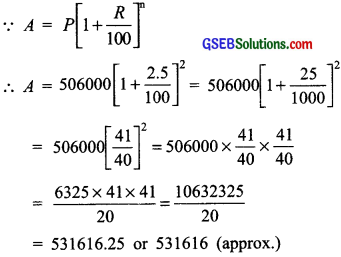= 53161625 or 531616 (approx.)
Thus, the count of bacteria after 2 hours will be 531616 (appiox.)

Question 12.
A scooter was bought at ₹ 42,000 has value depredated at the raie of 8% per annum. Find its value after one year?
Solution:
Initial cost (value) of the scooter (P) = ₹ 42000
Depreciation rate = 8% p.a.
Time = 1 year ⇒ n = 1
Using A = P[1 + $$\frac{R}{100}$$]n, we have
A = ₹ 42000 × [1 – $$\frac{8}{100}$$]1
= ₹ 42000 × $$\frac{92}{100}$$ = ₹ 420 × 92 = ₹ 38640
Thus, the value of the scooter after 1 year will be ₹ 38640.